# 1. Partial Least Squares (PLS) Models

Laboratory for Bioinformatics Research, RIKEN Center for Biosystems Dynamics Research
[email protected]

# Introduction

In this vignette, we consider a supervised dimensional reduction method partial least squares (PLS).

Test data is available from toyModel.

library("guidedPLS")
data <- guidedPLS::toyModel("Easy")
str(data, 2)
## List of 8
##  $X1 : int [1:100, 1:300] 86 101 95 106 113 85 88 103 106 84 ... ##$ X2      : int [1:200, 1:150] 106 81 91 101 91 105 111 81 113 105 ...
##  $Y1 : int [1:100, 1:50] 101 77 77 87 101 89 111 113 101 112 ... ##$ Y1_dummy: num [1:100, 1:3] 1 1 1 1 1 1 1 1 1 1 ...
##  $Y2 : int [1:200, 1:50] 107 81 102 90 84 106 97 90 88 115 ... ##$ Y2_dummy: num [1:200, 1:3] 1 1 1 1 1 1 1 1 1 1 ...
##  $col1 : chr [1:100] "#66C2A5" "#66C2A5" "#66C2A5" "#66C2A5" ... ##$ col2    : chr [1:200] "#66C2A5" "#66C2A5" "#66C2A5" "#66C2A5" ...

You will see that there are three blocks in the data matrix as follows.

suppressMessages(library("fields"))
layout(c(1,2,3))
image.plot(data$Y1_dummy, main="Y1 (Dummy)", legend.mar=8) image.plot(data$Y1, main="Y1", legend.mar=8)
image.plot(data$X1, main="X1", legend.mar=8)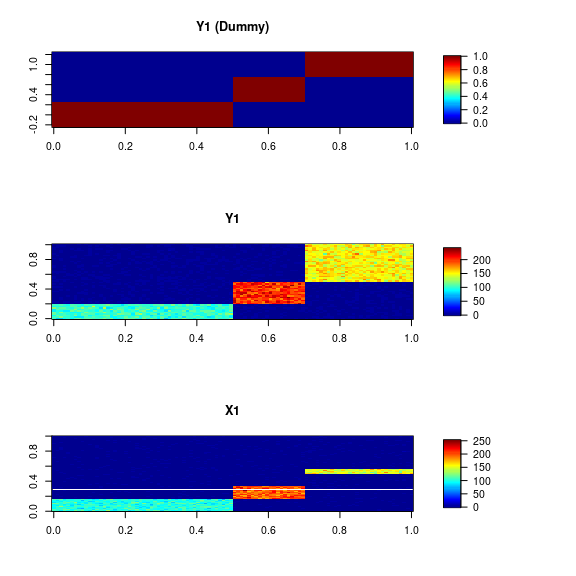# Partial Least Squares by Singular Value Decomposition (PLS-SVD) Here, suppose that we have two data matrices $$X$$ ($$N \times M$$) and $$Y$$ ($$N \times L$$), and their column vectors are assumed to be centered. Considering the projection of them to lower dimension, we have the scores $$XV$$ and $$YW$$, where $$V$$ ($$M \times K$$) and $$W$$ ($$L \times K$$) are called loading matrices for $$X$$ and $$Y$$, respectively. Depending on the loading matrice, these scores can take various values, but consider $$V$$ and $$W$$ such that the covariance is maximized as follows: $\max_{V,W} \mathrm{tr} \left( V^{T}X^{T}YW \right)\ \mathrm{s.t.}\ V^{T}V = W^{T}W = I_{K}$ This is known as Partial Least Squares (PLS). The widely used PLS algorithms are NIPALS and SIMPLS but here we introduce more simple one, Partial Least Squares by Singular Value Decomposition (PLS-SVD (Lê Cao 2008)). PLS-SVD is solved by svd against cross-product matrix $$X'Y$$. This formulation is also known as Guided Principal Component Analysis (guided-PCA (Reese S E 2013)). ## Basic Usage PLSSVD is performed as follows. out1 <- PLSSVD(X=data$X1, Y=data$Y1, k=2) plot(out1$scoreX, col=data$col1, pch=16)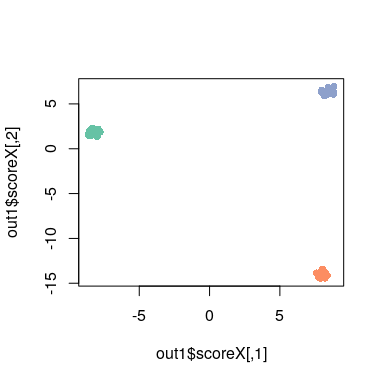Optionally, deflation mode, in which each loading and score vector is calculated incrementally, not at once, can be applied as follows. out2 <- PLSSVD(X=data$X1, Y=data$Y1, k=2, deflation=TRUE) plot(out2$scoreX, col=data$col1, pch=16)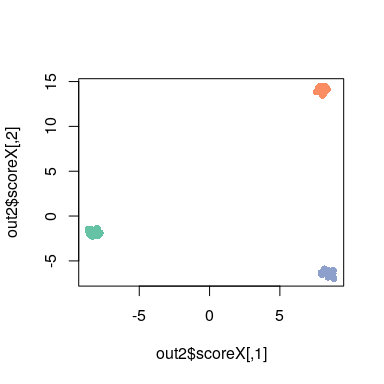This mode is often used to make the column vectors of a score matrix orthogonal to each other. # Partial Least Squares Discriminant Analysis (PLS-DA) In many cases, $$X$$ and $$Y$$ in PLS are assumed to be continuous. On the other hand, some matrices containing discrete values may be set for $$Y$$. For example, a dummy variable matrix containing only the values 0 and 1, which means the correspondence of data and group in $$X$$, is used as $$Y$$. Such a formulation is called Partial Least Squares Discriminant Analysis (PLS-DA (Lê Cao 2008)). In the context of distinguishing it from PLS-DA, the former is called Partial Least Squares Regression (PLS-R). ## Basic Usage PLS-DA by PLSSVD is performed as follows. out3 <- PLSSVD(X=data$X1, Y=data$Y1_dummy, k=2, fullrank=TRUE) plot(out3$scoreX, col=data$col1, pch=16)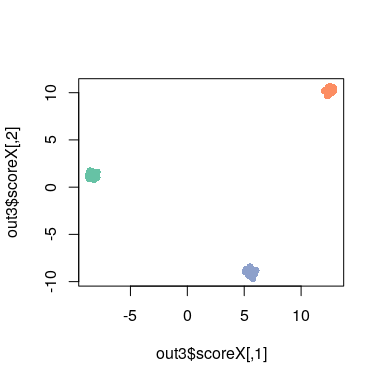# Sparse Partial Least Squares Discriminant Analysis (sPLS-DA) Sparse Partial Least Squares Discriminant Analysis (sPLS-DA (Lê Cao 2008)) is an extension of PLS-DA to enhance the interpretability of the scores and loadings by making the values sparse. Soft-thresholding function forces the values within a certain range ($$-\lambda$$ to $$\lambda$$) to be adjusted to zero. ## Basic Usage sPLSDA is performed as follows. Unlike PLSDA, sPLSDA has a lambda parameter that controls the degree of sparsity of the solution: out4 <- sPLSDA(X=data$X1, Y=data$Y1_dummy, k=2, lambda=30) The scores look almost identical to those of PLSDA: plot(out4$scoreX, col=data$col1, pch=16)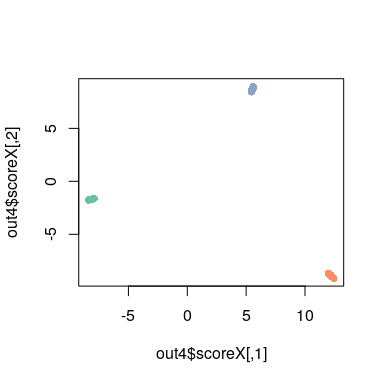However, you will see that the solution (loading) is sparser than PLSDA: layout(cbind(1:2, 3:4)) barplot(out3$loadingX[,1], main="PLSDA (1st loading)")
barplot(out3$loadingX[,2], main="PLSDA (2nd loading)") barplot(out4$loadingX[,1], main="sPLSDA (1st loading)")
barplot(out4$loadingX[,2], main="sPLSDA (2nd loading)")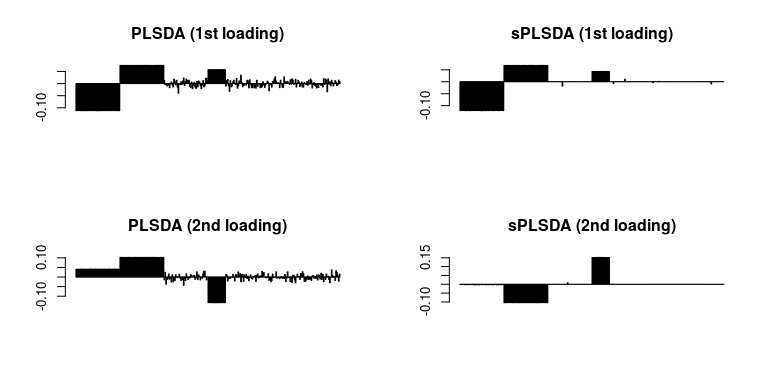# Comparison with unsupervised learning Next, we use another data data2. data2 <- guidedPLS::toyModel("Hard") str(data2, 2) ## List of 8 ##$ X1      : int [1:100, 1:300] 81 110 109 117 98 108 104 89 87 103 ...
##  $X2 : int [1:200, 1:150] 103 118 97 88 116 99 87 97 79 108 ... ##$ Y1      : int [1:100, 1:50] 89 119 104 96 92 100 98 99 107 87 ...
##  $Y1_dummy: num [1:100, 1:3] 1 1 1 1 1 1 1 1 1 1 ... ##$ Y2      : int [1:200, 1:50] 111 109 96 97 108 104 121 112 102 82 ...
##  $Y2_dummy: num [1:200, 1:3] 1 1 1 1 1 1 1 1 1 1 ... ##$ col1    : chr [1:100] "#66C2A5" "#66C2A5" "#66C2A5" "#66C2A5" ...
##  $col2 : chr [1:200] "#66C2A5" "#66C2A5" "#66C2A5" "#66C2A5" ... data2 has some blocks at the same coordinates as data in the matrix, but the background noise is larger and the block values are set smaller than data, resulting in a low S/N ratio like below. layout(t(1:2)) image.plot(data$X1, main="X1", legend.mar=8)
image.plot(data2$X1, main="X1 (Noisy)", legend.mar=8)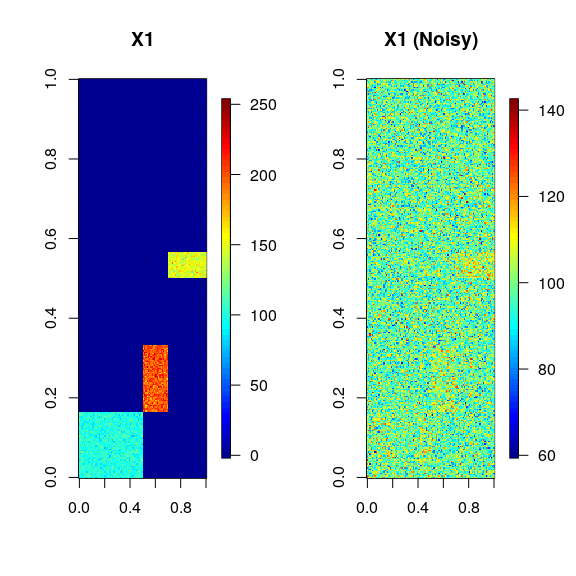For such data, supervised learning has difficulty extracting the signal from the noise. For example, let’s perform an unsupervised learning method principal component analysis (PCA) using only $$X$$. out.pca <- prcomp(data2$X1, center=TRUE, scale=FALSE)

Next, perform PLS-DA, which is a supervised learning using $$X$$, and label information $$Y$$ as well.

out5 <- PLSSVD(X=data2$X1, Y=data2$Y1_dummy, k=2, fullrank=TRUE)

Comparing the two, it is clear that PLS-DA, which uses labels, captures the structure of the data better like below.

layout(t(1:2))
plot(out.pca$x[, 1:2], col=data2$col1, main="PCA", pch=16)
plot(out5$scoreX, col=data2$col1, main="PLS-DA", pch=16)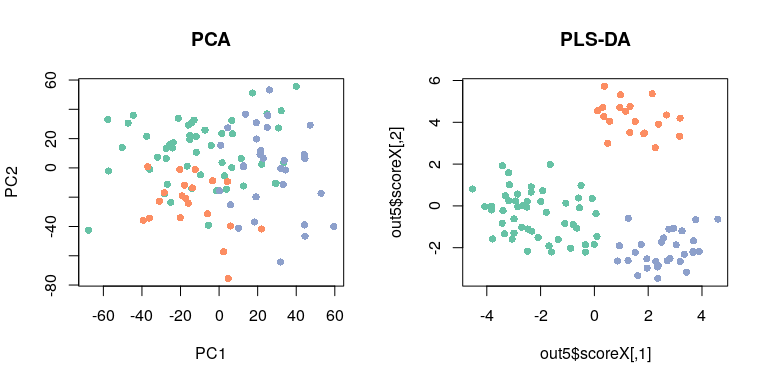Let’s compare the two with the PLS-DA loading performed on data. In PCA loading, PC1 correlates well with PLS-DA loading, but the correlation is a bit smaller for PC2. In contrast, in PLS-DA (Noisy), the first and second loadings correlate well with the PLS-DA loadings. In other words, the use of labels mitigates the effect of noise.

layout(t(1:2))
image.plot(cor(out5$scoreX, out.pca$x[,1:2]),
xlab="PLS-DA (Noisy)", ylab="PCA (Noisy)", legend.mar=8)
image.plot(cor(out5$scoreX, out3$scoreX),
xlab="PLS-DA (Noisy)", ylab="PLS-DA", legend.mar=8)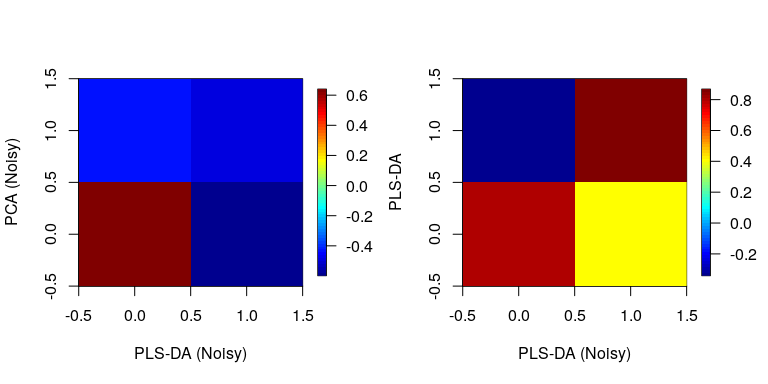# Session Information

## R version 4.3.0 (2023-04-21)
## Platform: x86_64-pc-linux-gnu (64-bit)
## Running under: Debian GNU/Linux bookworm/sid
##
## Matrix products: default
## LAPACK: /usr/lib/x86_64-linux-gnu/openblas-pthread/libopenblasp-r0.3.21.so;  LAPACK version 3.11.0
##
## locale:
##   LC_CTYPE=en_US.UTF-8       LC_NUMERIC=C
##   LC_TIME=en_US.UTF-8        LC_COLLATE=en_US.UTF-8
##   LC_MONETARY=en_US.UTF-8    LC_MESSAGES=en_US.UTF-8
##   LC_PAPER=en_US.UTF-8       LC_NAME=C
##  LC_MEASUREMENT=en_US.UTF-8 LC_IDENTIFICATION=C
##
## time zone: Etc/UTC
## tzcode source: system (glibc)
##
## attached base packages:
##  stats     graphics  grDevices utils     datasets  methods   base
##
## other attached packages:
##  fields_14.1       viridis_0.6.2     viridisLite_0.4.1 spam_2.9-1
##  guidedPLS_1.0.0
##
## loaded via a namespace (and not attached):
##   Matrix_1.5-3     gtable_0.3.3     jsonlite_1.8.4   highr_0.10
##   compiler_4.3.0   maps_3.4.1       gridExtra_2.3    jquerylib_0.1.4
##   scales_1.2.1     yaml_2.3.7       fastmap_1.1.1    lattice_0.20-45
##  ggplot2_3.4.2    R6_2.5.1         knitr_1.42       dotCall64_1.0-2
##  tibble_3.2.1     munsell_0.5.0    bslib_0.4.2      pillar_1.9.0
##  rlang_1.1.0      utf8_1.2.3       cachem_1.0.7     xfun_0.39
##  sass_0.4.5       cli_3.6.1        magrittr_2.0.3   digest_0.6.31
##  grid_4.3.0       irlba_2.3.5.1    lifecycle_1.0.3  vctrs_0.6.2
##  evaluate_0.20    glue_1.6.2       fansi_1.0.4      colorspace_2.1-0
##  rmarkdown_2.21   tools_4.3.0      pkgconfig_2.0.3  htmltools_0.5.5